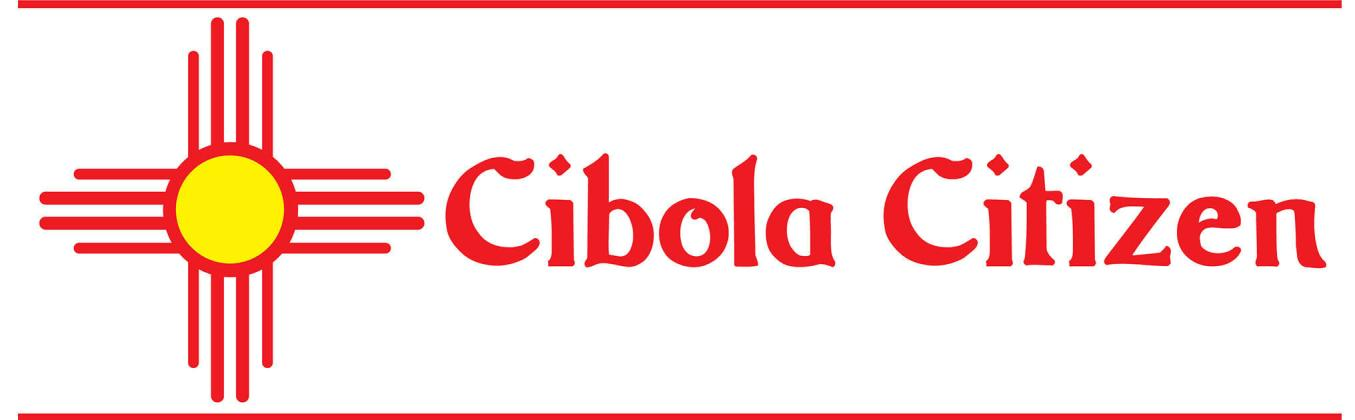# COVID -19

•The New Mexico Department of Health posts daily updates,https://cv.nmhealth.org/ Statistics are listed below by U.S. Postal Service zip codes, Cibola County. Tuesday, Oct. 13 87005 = 6 87007 = 12 87014 = 72 87020 = 192 87021 = 29 87026 = 103 87034 = 24 87038 = 17 87040 = 6 87049 = 21 87051 = 15 87315 = 0 87357 = 35 State totals for this date: 33,713 positive tests, 918 deaths, 1…